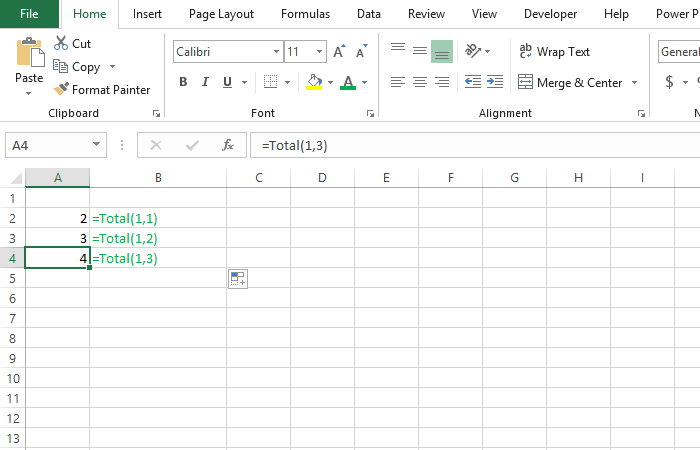# Custom functions

In the preceding text we talked about procedures and now we will mention functions. Functions are known as subroutines that calculate and return certain values. We can call them from other programs and it is possible to create custom functions that we will later use in worksheets. This is useful when Excel does not have the specific features you need to perform everyday work.

We create a new function in a similar way to the way subroutines are created, and the syntax is as follows:

Function <function name> [(<argument 1>, …, <argument n>] As <data type>

End Function

Behind the name of the function in parentheses, arguments should be given, if they exist. Otherwise, we will use “empty brackets”. Behind them, it is necessary, similar to the declaration of variables, to indicate the type of value that the function returns. The last line of code in the function body should be the name of the function followed by the equality sign and the value it returns.

Let’s write a simple Total function to calculate a set of two given integers:

Function Total (a As Integer, As Integer) As Integer
Total = a + b
End Function

If we now return to Excel, we position it in a cell, and then start with the formula we will be offered and the function we created ourselves. It is necessary to set two input arguments and it will return their total.